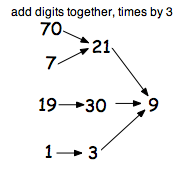Follow some numbers and see where they go.

The simple rule is: choose a number, add the digits together then multiply by 2 and then do the same again.

Suppose you start with 56 then you get 5+6 = 11 and 11 x 2 =22 so the next number is 22. Carry on and you get: 56, 22, 8, 16, 14, 10, 2, 4, 8, 16, 14, 10, 2, …